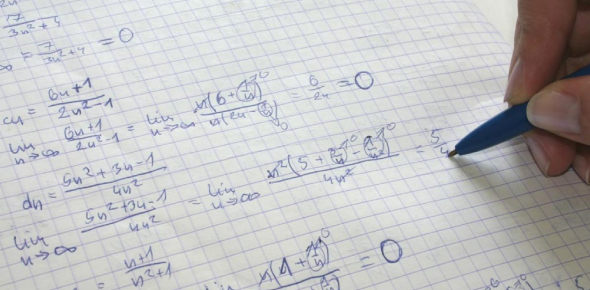# Math Practice Test: MCQ Quiz!

10 Questions | Total Attempts: 777SettingsHey there folks! How are you? Do you play with numbers? Do you solve mathematical calculations in minutes? If you're a genius, then do take this quiz to test your memory and calculation skills. There is a list of 10 random Math questions that you have to solve without using the calculator. Each question has a 2 minutes time limit. Let's try it out.

• 1.
How many lines can be drawn through two points?
• A.

1

• B.

2

• C.

3

• D.

Not possible

• 2.
Factors of 9 are…..
• A.

1, 2 and 3

• B.

1, 2, 3 and 9

• C.

1, 6 and 9

• D.

None of these.

• 3.
When we multiply an exact number by zero, what will be the exact answer?
• A.

The exact number

• B.

Cannot be multiplied

• C.

Zero

• D.

None of these

• 4.
How many digits answers will we get when we add 99 and 1?
• A.

1

• B.

3

• C.

99

• D.

100

• 5.
What are 999 times 100.0?
• A.

199.0

• B.

999.0

• C.

9990

• D.

99900

• 6.
1010 gram = ________kg.
• A.

10.10 kg

• B.

101.0 kg.

• C.

1.001 kg.

• D.

1.01 kg.

• 7.
Simplify: {36 ÷ (-9)} ÷ {(-24) ÷ 6}
• A.

1

• B.

2

• C.

3

• D.

-1

• 8.
Simplify: 0 × 102
• A.

10

• B.

10.2

• C.

102

• D.

None of these.

• 9.
Subtract - 8a from - 3a
• A.

2a

• B.

5a

• C.

- 11a

• D.

11a

• 10.
The average of three person’s age is 9 years. Find the sum of their age.
• A.

18

• B.

21

• C.

24

• D.

27

Related TopicsBack to top